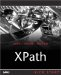Creating Conditional Expressions

As we saw in the overview in Chapter 7, XPath 2.0 supports a conditional expression that uses the keywords if , then , and else . Here's what this expression, also called the if expression, looks like in its general form:

if  expression  then  then-expression  else  else-expression

The expression following the if keyword is called the test expression , and the expressions following the then and else keywords are called the then-expression and else-expression , respectively.

If the value of the test expression is true, the value of the then-expression is returned. If the Boolean value of the test expression is false, the value of the else-expression is returned.

Here's an example using a conditional expression in an XSLT 2.0 stylesheet. In this case, we'll declare an XSLT variable named \$temperature that holds the value 80:

<xsl:variable name="temperature" select="80" />

Now we'll test that new variable's value in a conditional expression, evaluating to the text "Too hot" if the temperature is above 72, and "OK" otherwise :

if (\$temperature > 72) then 'Too hot' else 'OK'

You can see what the complete XSLT 2.0 stylesheet looks like in ch08_03.xsl (Listing 8.3).

Listing 8.3 An XSLT Example Using the if Expression ( ch08_03.xsl )
<xsl:stylesheet version="2.0"     xmlns:xsl="http://www.w3.org/1999/XSL/Transform"     xmlns:xs="http://www.w3.org/2001/XMLSchema"> <xsl:variable name="temperature" select="80" />  <xsl:template match="/">   <xsl:value-of select="if (\$temperature > 72) then 'Too hot' else 'OK'"/>   </xsl:template>  </xsl:stylesheet>

And here's the result you get when you use Saxon:

<?xml version="1.0" encoding="UTF-8"?> Too hot

You can use any kind of a test expression, as long as it evaluates to a true / false value. For example, in this case, we're checking which of two planets has the greater mass, and returning the one that does:

if (//planet/mass > //planet/mass)     then //planet     else //planet

You don't have to compare values either; you can simply test for the existence of an item, as here, where we're testing if a <planet> element has a <name> child element:

if (//planet/name)     then //planet/name     else //planet/name

You can also nest if expressions, as in this example:

if (\$fruit eq "apple") then "It's an apple." else if (\$fruit eq "orange") then "It's an orange." else "I have no idea what this is."XPath Kick Start: Navigating XML with XPath 1.0 and 2.0
ISBN: 0672324113
EAN: 2147483647
Year: 2002
Pages: 131

Similar book on Amazon